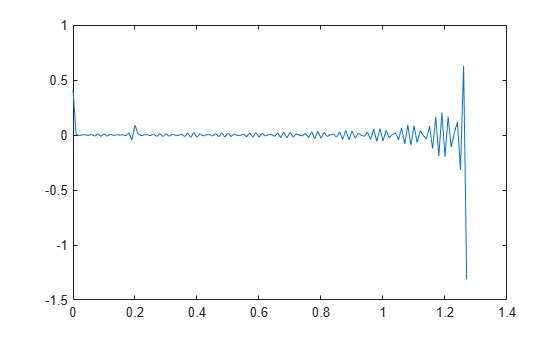# Cepstrum Analysis

### What Is a Cepstrum?

Cepstrum analysis is a nonlinear signal processing technique with a variety of applications in areas such as speech and image processing.

The complex cepstrum of a sequence x is calculated by finding the complex natural logarithm of the Fourier transform of x, then the inverse Fourier transform of the resulting sequence:

`$\underset{}{\overset{ˆ}{x}}=\frac{1}{2\pi }{\int }_{-\pi }^{\pi }\mathrm{log}\left[X\left({e}^{j\omega }\right)\right]{e}^{j\omega n}\phantom{\rule{0.16666666666666666em}{0ex}}d\omega .$`

The toolbox function `cceps` performs this operation, estimating the complex cepstrum for an input sequence. It returns a real sequence the same size as the input sequence.

Try using cceps in an echo detection application. First, create a 45 Hz sine wave sampled at 100 Hz. Add an echo of the signal, with half the amplitude, 0.2 seconds after the beginning of the signal.

```t = 0:0.01:1.27; s1 = sin(2*pi*45*t); s2 = s1 + 0.5*[zeros(1,20) s1(1:108)];```

Compute and plot the complex cepstrum of the new signal.

```c = cceps(s2); plot(t,c)```The complex cepstrum shows a peak at 0.2 seconds, indicating the echo.

The real cepstrum of a signal x, sometimes called simply the cepstrum, is calculated by determining the natural logarithm of magnitude of the Fourier transform of x, then obtaining the inverse Fourier transform of the resulting sequence:

`${c}_{x}=\frac{1}{2\pi }{\int }_{-\pi }^{\pi }\mathrm{log}|X\left({e}^{j\omega }\right)|{e}^{j\omega n}\phantom{\rule{0.16666666666666666em}{0ex}}d\omega .$`

The toolbox function `rceps` performs this operation, returning the real cepstrum for a sequence. The returned sequence is a real-valued vector the same size as the input vector.

The `rceps` function also returns a unique minimum-phase sequence that has the same real cepstrum as the input. To obtain both the real cepstrum and the minimum-phase reconstruction for a sequence, use `[y,ym] = rceps(x)`, where `y` is the real cepstrum and `ym` is the minimum phase reconstruction of `x`. The following example shows that one output of `rceps` is a unique minimum-phase sequence with the same real cepstrum as `x`.

```y = [4 1 5]; % Non-minimum phase sequence [xhat,yhat] = rceps(y); xhat2 = rceps(yhat); [xhat' xhat2']```
```ans = 3×2 1.6225 1.6225 0.3400 0.3400 0.3400 0.3400 ```

### Inverse Complex Cepstrum

To invert the complex cepstrum, use the `icceps` function. Inversion is complicated by the fact that the `cceps` function performs a data-dependent phase modification so that the unwrapped phase of its input is continuous at zero frequency. The phase modification is equivalent to an integer delay. This delay term is returned by `cceps` if you ask for a second output:

```x = 1:10; [xhat,delay] = cceps(x)```
```xhat = 1×10 2.2428 -0.0420 -0.0210 0.0045 0.0366 0.0788 0.1386 0.2327 0.4114 0.9249 ```
```delay = 1 ```

To invert the complex cepstrum, use `icceps` with the original delay parameter:

`icc = icceps(xhat,2)`
```icc = 1×10 2.0000 3.0000 4.0000 5.0000 6.0000 7.0000 8.0000 9.0000 10.0000 1.0000 ```

As shown in the above example, with any modification of the complex cepstrum, the original delay term may no longer be valid. You will not be able to invert the complex cepstrum exactly.